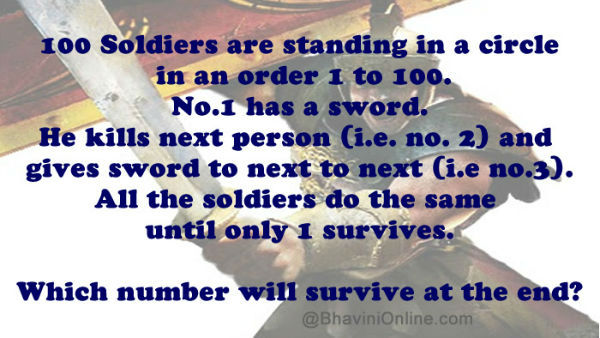# Josephus Problem: 100 Soldiers in a Circle Riddle

A popular riddle which you should attempt if you have never came across it.

100 Soldiers are standing in a circle in an order 1 to 100.

No.1 has a sword.

He kills next person (i.e. no. 2) and gives sword to next to next (i.e no.3).

All the soldiers do the same until only 1 survives.

Which number will survive at the end?So were you able to solve the riddle? Leave your answers in the comment section below.

## 5 Replies to “Josephus Problem: 100 Soldiers in a Circle Riddle”

1. ashish modi says:

import java.io.*;
import java.util.*;

public class Solution
{
public static void main(String[] args)
{
int a,b;
Scanner sc=new Scanner(System.in);
a=sc.nextInt();
b=a;
int[] arr= new int;
int index=0;
int[] ar= new int;
int[] arrr= new int;
while(b > 0)
{
arr[index++] = b%2;
b = b/2;
}
for(int k=0;k<index;k++)
{
ar[k]=arr[index-k-1];
}
for(int l=0;l<index-1;l++)
{
arrr[l]=ar[l+1];
}
arrr[index-1]=ar;
int total=0;
for(int g = 0; g < index; g++)
{
total += Math.pow(2,g) * arrr[index – g – 1];
}
System.out.println(total);

}
}

2. Shiv says:

I got the Josephus problem…but 73 is the correct answer because they wanted to suicide but if we see the riddle here…9, 41, and 73 will be in the last round but if they are forced to do this…then 9 will kill 41 but won’t give the sword to 73..just to kill himself…i mean that way 9 could be the survivor. I mean i got the mathematics but actually didnt get the logic…plz someone explain..

3. Raphael Winkler says:

at the end of the second round wouldnt number 97 be left with the sword? and therefore have to kill number 1?

4. Nillan says:

How we got 64 out of that? And what is Answer of 1000 men?

1. Hi Nilan,
You can google for “Josephus Problem” for further explanation on the theory.
It’s an interesting concept but needs deeper reading.
Thanks